# Assigning Points based on Late Time in Google Sheets

It is shown in this lesson how to utilize the IF function in Excel to allocate points based on the amount of time that has passed.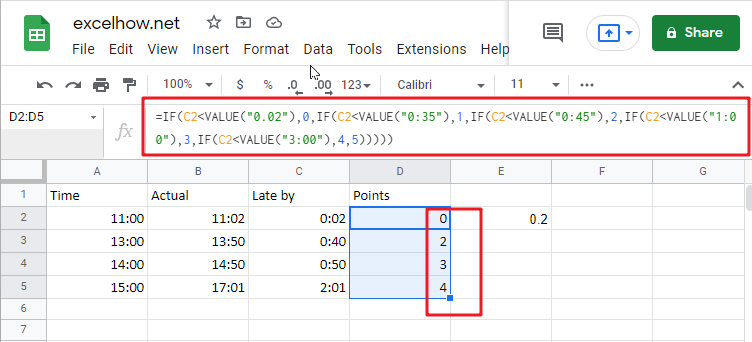## Generic formula:

`=IF(C2<VALUE("0:2"),0,IF(C2<VALUE("0:35"),1,IF(C2<VALUE("0:45"),2,IF(C2<VALUE("1:00"),3,IF(C2<VALUE("3:00"),4,5)))))`

## Return value

This formula provides the number of points that have been assigned depending on the late time.

## How this formula work

For example, below is a list of point regulations and time records; kindly use the formula below to find the appropriate rule or record.

`=IF(C2<VALUE("0:2"),0,IF(C2<VALUE("0:35"),1,IF(C2<VALUE("0:45"),2,IF(C2<VALUE("1:00"),3,IF(C2<VALUE("3:00"),4,5)))))`

A pop-up with further information will appear if you press the Enter key.

To use this formula, just drag the fill handles over the cells.

##IF function is being used to evaluate for defined criteria and then returns the results that match those circumstances. Explain the formula in five parts, as follows:

`IF (late_by_time<VALUE ("0:2"),0,` It returns 0 if the late time is much less than 2 minutes.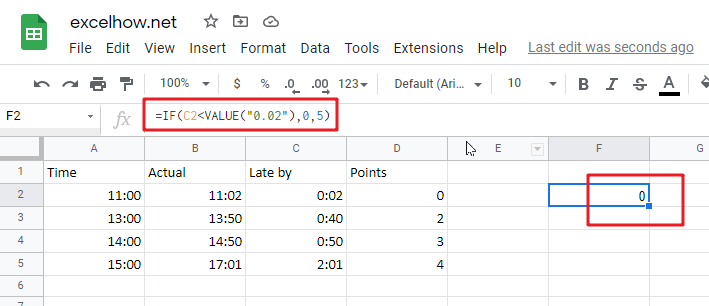`IF (late_by_time <VALUE ("0:35"),1,` The function returns 1 if the late time is much less than 35 minutes but higher than or equivalent to 2 minutes.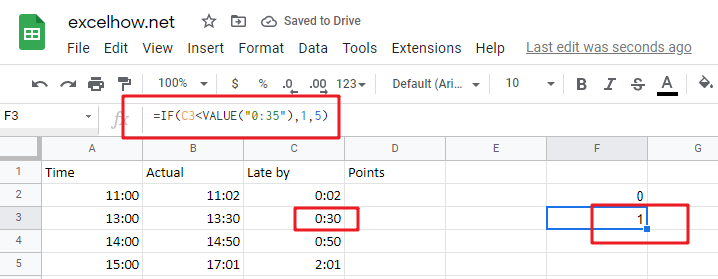`IF (late_by_time <VALUE ("0:45"),2`, If the late time is even less than 45 minutes but higher than or equivalent to 35 mins, it returns 2.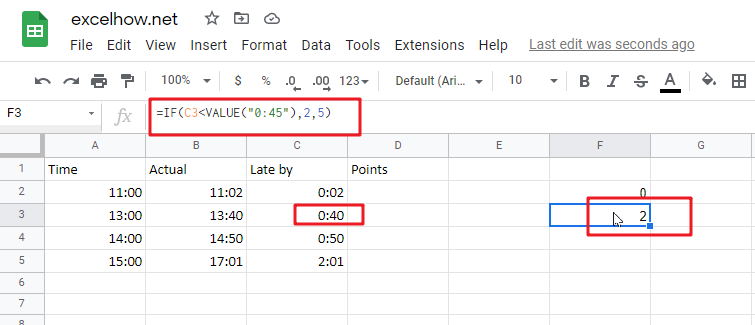`IF (late_by_time <VALUE ("1:00"),3`, If the late time is even less than 60 minutes but higher than or equivalent to 45 mins, it returns 3.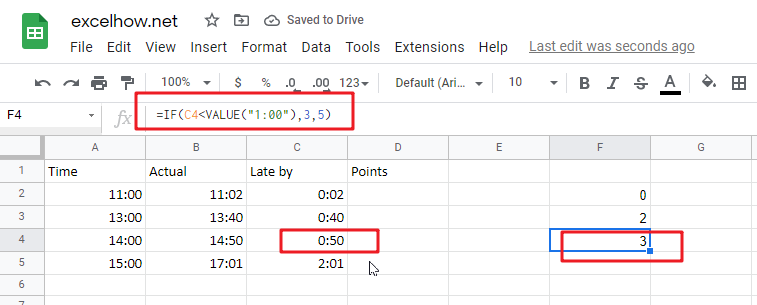`IF (late_by_time <VALUE ("3:00"),4`, The result is 4 if the late time is less than 3 hours but larger than or equivalent to 60 minutes.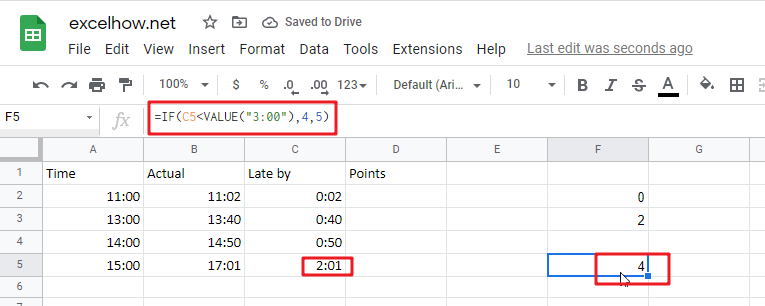5))))) This function returns five if the late time is more than or equal to three hours.

When converting text to numbers, the value function is employed. It does this by converting the text time to a number.